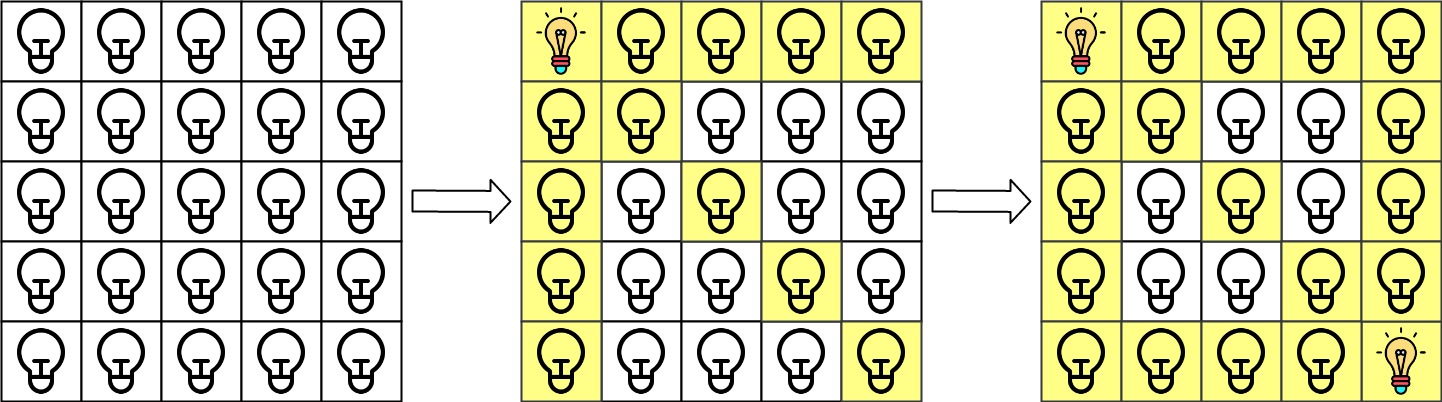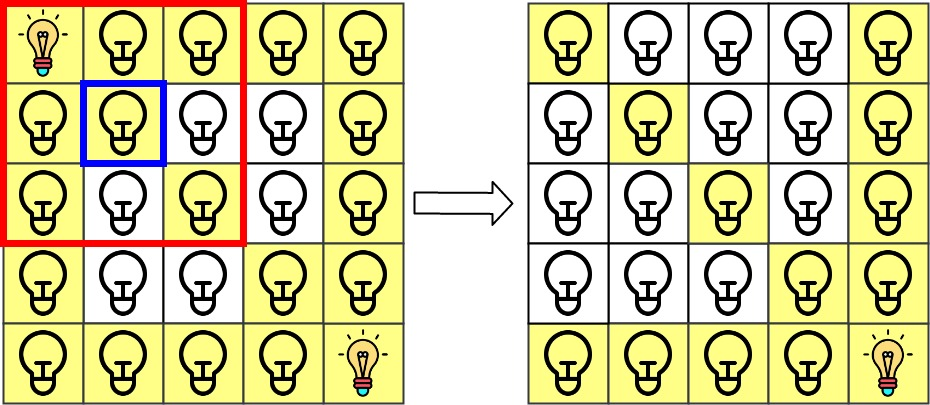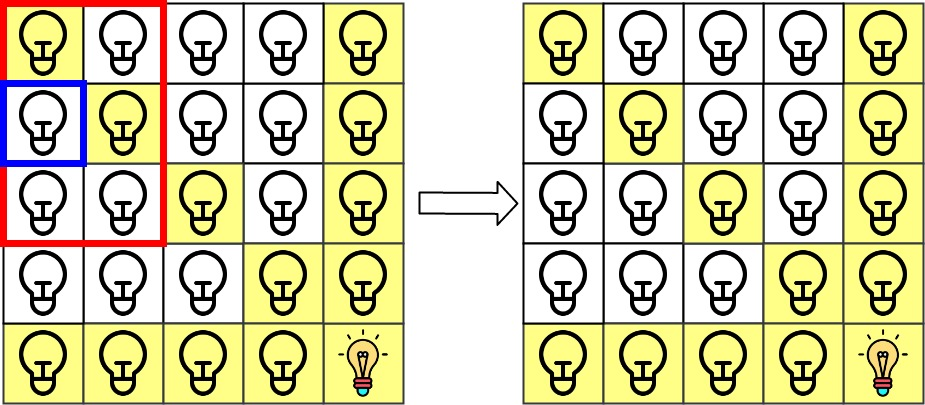| English | 简体中文 |

# 1001. Grid Illumination

## Description

There is a 2D grid of size n x n where each cell of this grid has a lamp that is initially turned off.

You are given a 2D array of lamp positions lamps, where lamps[i] = [rowi, coli] indicates that the lamp at grid[rowi][coli] is turned on. Even if the same lamp is listed more than once, it is turned on.

When a lamp is turned on, it illuminates its cell and all other cells in the same row, column, or diagonal.

You are also given another 2D array queries, where queries[j] = [rowj, colj]. For the jth query, determine whether grid[rowj][colj] is illuminated or not. After answering the jth query, turn off the lamp at grid[rowj][colj] and its 8 adjacent lamps if they exist. A lamp is adjacent if its cell shares either a side or corner with grid[rowj][colj].

Return an array of integers ans, where ans[j] should be 1 if the cell in the jth query was illuminated, or 0 if the lamp was not.

Example 1:Input: n = 5, lamps = [[0,0],[4,4]], queries = [[1,1],[1,0]]
Output: [1,0]
Explanation: We have the initial grid with all lamps turned off. In the above picture we see the grid after turning on the lamp at grid then turning on the lamp at grid.
The 0th query asks if the lamp at grid is illuminated or not (the blue square). It is illuminated, so set ans = 1. Then, we turn off all lamps in the red square.The 1st query asks if the lamp at grid is illuminated or not (the blue square). It is not illuminated, so set ans = 0. Then, we turn off all lamps in the red rectangle.Example 2:

Input: n = 5, lamps = [[0,0],[4,4]], queries = [[1,1],[1,1]]
Output: [1,1]


Example 3:

Input: n = 5, lamps = [[0,0],[0,4]], queries = [[0,4],[0,1],[1,4]]
Output: [1,1,0]


Constraints:

• 1 <= n <= 109
• 0 <= lamps.length <= 20000
• 0 <= queries.length <= 20000
• lamps[i].length == 2
• 0 <= rowi, coli < n
• queries[j].length == 2
• 0 <= rowj, colj < n